• +91 9971497814
• info@interviewmaterial.com

# RD Chapter 24- The Circle Ex-24.1 Interview Questions Answers

### Related Subjects

Question 1 :
Find the equation of the circle with:
(i) Centre (-2, 3) and radius 4.
(ii) Centre (a, b) and radius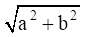.
(iii) Centre (0, – 1) and radius 1.
(iv) Centre (a cos α, a sin α) and radius a.
(v) Centre (a, a) and radius √2 a.

(i) Centre (-2, 3) andradius 4.

Given:

The radius is 4 andthe centre (-2, 3)

By using the formula,

The equation of thecircle with centre (p, q) and radius ‘r’ is (x – p)2 + (y – q)2 =r2

Where, p = -2, q = 3,r = 4

Now by substitutingthe values in the above equation, we get

(x – p)2 +(y – q)2 = r2

(x – (-2))2 +(y – 3)2 = 42

(x + 2)2 +(y – 3)2 = 16

x2 +4x + 4 + y2 – 6y + 9 = 16

x2 + y2 +4x – 6y – 3 = 0

The equation of thecircle is x2 + y2 + 4x – 6y – 3 = 0.

Given:and the centre (a, b)

By using the formula,

The equation of thecircle with centre (p, q) and radius ‘r’ is (x – p)2 + (y – q)2 =r2

Where, p = a, q = b, r=Now by substitutingthe values in the above equation, we get

(x – p)2 +(y – q)2 = r2

(x – a)2 +(y – b)2 =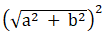x2 –2ax + a2 + y2 – 2by + b2 = a2 +b2

x2 + y2 –2ax – 2by = 0

The equation of thecircle is x2 + y2 – 2ax – 2by = 0

(iii) Centre (0, -1) andradius 1.

Given:

The radius is 1 andthe centre (0, -1)

By using the formula,

The equation of thecircle with centre (p, q) and radius ‘r’ is (x – p)2 + (y – q)2 =r2

Where, p = 0, q = -1,r = 1

Now by substitutingthe values in the above equation, we get

(x – p)2 +(y – q)2 = r2

(x – 0)2 +(y – (-1))2 = 12

(x – 0)2 +(y + 1)2 = 1

x2 + y2 +2y + 1 = 1

x2 + y2 +2y = 0

The equation of thecircle is x2 + y2 + 2y = 0.

(iv) Centre (a cos α, a sinα) and radius a.

Given:

The radius is ‘a’ andthe centre (a cos α, a sin α)

By using the formula,

The equation of thecircle with centre (p, q) and radius ‘r’ is (x – p)2 + (y – q)2 =r2

Where, p = a cos α, q= a sin α, r = a

Now by substitutingthe values in the above equation, we get

(x – p)2 +(y – q)2 = r2

(x – a cosα)2 +(y – a sinα)2 = a2

x2 –(2acosα)x + a2cos2α + y2 – (2asinα)y + a2sin2α= a2

We know that sin2θ+ cos2θ = 1

So,

x2 –(2acosα)x + y2 – 2asinαy + a2 = a2

x2 + y2 –(2acosα)x – (2asinα)y = 0

The equation of thecircle is x2 + y2 – (2acosα) x – (2asinα) y =0.

(v) Centre (a, a) andradius √2 a.

Given:

The radius is√2 a and the centre (a, a)

By using the formula,

The equation of thecircle with centre (p, q) and radius ‘r’ is (x – p)2 + (y – q)2 =r2

Where, p = a, q = a, r= √2 a

Now by substitutingthe values in the above equation, we get

(x – p)2 +(y – q)2 = r2

(x – a)2 +(y – a)2 = (√2 a)2

x2 –2ax + a2 + y2 – 2ay + a2 = 2a2

x2 + y2 –2ax – 2ay = 0

The equation of thecircle is x2 + y2 – 2ax – 2ay = 0.

Question 2 :

Find the centre and radius of each of the following circles:
(i) (x – 1)2 + y2 = 4

(ii) (x + 5)2 + (y + 1)2 = 9

(iii) x2 + y2 – 4x + 6y = 5

(iv) x2 + y2 – x + 2y – 3 = 0

(i) (x – 1)2 +y2 = 4

Given:

The equation (x – 1)2 +y2 = 4

We need to find thecentre and the radius.

By using the standardequation formula,

(x – a)2 +(y – b)2 = r2 …. (1)

Now let us convertgiven circle’s equation into the standard form.

(x – 1)2 +y2 = 4

(x – 1)2 +(y – 0)2 = 22 ….. (2)

By comparing equation(2) with (1), we get

Centre = (1, 0) andradius = 2

The centre of thecircle is (1, 0) and the radius is 2.

(ii) (x + 5)2 +(y + 1)2 = 9

Given:

The equation (x + 5)2 +(y + 1)2 = 9

We need to find thecentre and the radius.

By using the standardequation formula,

(x – a)2 +(y – b)2 = r2 …. (1)

Now let us convertgiven circle’s equation into the standard form.

(x + 5)2 +(y + 1)2 = 9

(x – (-5))2 +(y – ( – 1))2 = 32 …. (2)

By comparing equation(2) with (1), we get

Centre = (-5, -1) andradius = 3

The centre of thecircle is (-5, -1) and the radius is 3.

(iii) x2 + y2 –4x + 6y = 5

Given:

The equation x2 +y2 – 4x + 6y = 5

We need to find thecentre and the radius.

By using the standardequation formula,

(x – a)2 +(y – b)2 = r2 …. (1)

Now let us convertgiven circle’s equation into the standard form.

x2 + y2 –4x + 6y = 5

(x2 –4x + 4) + (y2 + 6y + 9) = 5 + 4 + 9

(x – 2)2 +(y + 3)2 = 18

(x – 2)2 +(y – (-3))2 = (3√2)2 … (2)

By comparing equation(2) with (1), we get

Centre = (2, -3) andradius = 3√2

The centre of thecircle is (2, -3) and the radius is 3√2.

(iv) x2 + y2 –x + 2y – 3 = 0

Given:

The equation x2 +y2 – x + 2y – 3 = 0

We need to find thecentre and the radius.

By using the standardequation formula,

(x – a)2 +(y – b)2 = r2 …. (1)

Now let us convertgiven circle’s equation into the standard form.

x2 + y2 –x + 2y – 3 = 0

(x2 –x + ¼) + (y2 + 2y + 1) – 3 – ¼ – 1 = 0

(x – ½)2 +(y + 1)2 = 17/4 …. (2)

By comparing equation(2) with (1), we get

Centre = (½, – 1) andradius = √17/2

The centre of thecircle is (½, -1) and the radius is √17/2.

Question 3 : Find the equation of the circle whose centre is (1, 2) and which passes through the point (4, 6).

Given:

Centre is (1, 2) andwhich passes through the point (4, 6).

Where, p = 1, q = 2

We need to find theequation of the circle.

By using the formula,

(x – p)2 +(y – q)2 = r2

(x – 1)2 +(y – 2)2 = r2

It passes through thepoint (4, 6)

(4 – 1)2 +(6 – 2)2 = r2

32 + 42 =r2

9 + 16 = r2

25 = r2

r = √25

= 5

So r = 5 units

We know that the equationof the circle with centre (p, q) and having radius ‘r’ is given by: (x – p)2 +(y – q)2 = r2

By substitute thevalues in the above equation, we get

(x – 1)2 +(y – 2)2 = 52

x2 –2x + 1 + y2 – 4y + 4 = 25

x2 + y2 –2x – 4y – 20 = 0.

The equation of thecircle is x2 + y2 – 2x – 4y – 20 = 0.

Question 4 : Find the equation of the circle passing through the point of intersection of the lines x + 3y = 0 and 2x – 7y = 0 and whose centre is the point of intersection of the lines x + y + 1 = 0 and x – 2y + 4 = 0.

Let us find the pointsof intersection of the lines.

On solving the lines x+ 3y = 0 and 2x – 7y = 0, we get the point of intersection to be (0, 0)

On solving the lines x+ y + 1 and x – 2y + 4 = 0, we get the point of intersection to be (-2, 1)

We have circle withcentre (-2, 1) and passing through the point (0, 0).

We know that theradius of the circle is the distance between the centre and any point on theradius. So, we find the radius of the circle.

So, the equation is (x– p)2 + (y – q)2 = r2

Where, p = -2, q = 1

(x + 2)2 +(y – 1)2 = r2 …. (1)

Equation (1) passesthrough (0, 0)

So, (0 + 2)2 +(0 – 1)2 = r2

4 + 1 = a2

5 = r2

r = √5

We know that theequation of the circle with centre (p, q) and having radius ‘r’ is given by: (x– p)2 + (y – q)2 = r2

By substitute thevalues in the above equation, we get

(x – (-2))2 +(y – 1)2 = (√5)2

(x + 2)2 +(y – 1)2 = 5

x2 +4x + 4 + y2 – 2y + 1 = 5

x2 + y2 +4x – 2y = 0

The equation of thecircle is x2 + y2 + 4x – 2y = 0.

Question 5 : Find the equation of the circle whose centre lies on the positive direction of y – axis at a distance 6 from the origin and whose radius is 4.

It is given that thecentre lies on the positive y – axis at a distance of 6 from the origin, we getthe centre (0, 6).

We have a circle withcentre (0, 6) and having radius 4.

We know that theequation of the circle with centre (p, q) and having radius ‘r’ is given by: (x– p)2 + (y – q)2 = r2

Where, p = 0, q = 6, r= 4

Now by substitutingthe values in the equation, we get

(x – 0)2 +(y – 6)2 = 42

x2 + y2 –12y + 36 = 16

x2 + y2 –12y + 20 = 0.

The equation of thecircle is x2 + y2 – 12y + 20 = 0.

Question 6 : If the equations of two diameters of a circle are 2x + y = 6 and 3x + 2y = 4 and the radius is 10, find the equation of the circle.

It is given that thecircle has the radius 10 and has diameters 2x + y = 6 and 3x + 2y = 4.

We know that thecentre is the intersection point of the diameters.

On solving thediameters, we get the centre to be (8, -10).

We have a circle withcentre (8, -10) and having radius 10.

By using the formula,

We know that theequation of the circle with centre (p, q) and having radius ‘r’ is given by: (x– p)2 + (y – q)2 = r2

Where, p = 8, q = -10,r = 10

Now by substitutingthe values in the equation, we get

(x – 8)2 +(y – (-10))2 = 102

(x – 8)2 +(y + 10)2 = 100

x2 –16x + 64 + y2 + 20y + 100 = 100

x2 + y2 –16x + 20y + 64 = 0.

The equation of thecircle is x2 + y2 – 16x + 20y + 64 = 0.

Question 7 :
Find the equation of the circle
(i) which touches both the axes at a distance of 6 units from the origin.
(ii) Which touches x – axis at a distance of 5 from the origin and radius 6 units.
(iii) Which touches both the axes and passes through the point (2, 1).
(iv) Passing through the origin, radius 17 and ordinate of the centre is – 15.

(i) which touches both theaxes at a distance of 6 units from the origin.

A circle touchesthe axes at the points (±6, 0) and (0, ±6).

So, a circle has acentre (±6, ±6) and passes through the point (0, 6).

We know that theradius of the circle is the distance between the centre and any point on theradius. So, we find the radius of the circle.

So, the equation is (x– p)2 + (y – q)2 = r2

Where, p = 6, q = 6

(x – 6)2 +(y – 6)2 = r2 …. (1)

Equation (1) passesthrough (0, 6)

So, (0 – 6)2 +(6 – 6)2 = r2

36 + 0 = r2

r = √36

= 6

We know that theequation of the circle with centre (p, q) and having radius ‘r’ is given by: (x– p)2 + (y – q)2 = r2

Now by substitutingthe values in the equation, we get

(x ± 6)2 +(y ± 6)2 = (6)2

x±12x + 36 + y± 12y + 36 = 36

x2 + y±12x ± 12y + 36 = 0

The equation of thecircle is x2 + y± 12x ± 12y + 36 = 0.

(ii) Which touches x – axisat a distance of 5 from the origin and radius 6 units.

A circle touchesthe x – axis at the points (±5, 0).

Let us assume thecentre of the circle is (±5, a).

We have a circle withcentre (5, a) and passing through the point (5, 0) and having radius 6.

We know that theradius of the circle is the distance between the centre and any point on theradius. So, we find the radius of the circle.

So, the equation is (x– p)2 + (y – q)2 = r2

Where, p = 5, q = a

(x – 5)2 +(y – a)2 = r2 …. (1)

Equation (1) passesthrough (5, 0)

So, (5 – 5)2 +(0 – 6)2 = r2

0 + 36 = r2

r = √36

= 6

We have got the centreat (±5, ±6) and having radius 6 units.

We know that theequation of the circle with centre (p, q) and having radius ‘r’ is given by: (x– p)2 + (y – q)2 = r2

Now by substitutingthe values in the equation, we get

(x ± 5)2 +(y ± 6)2 = (6)2

x2 ±10x + 25 + y2 ± 12y + 36 = 36

x2 + y2 ±10x ± 12y + 25 = 0.

The equation of thecircle is x2 + y2 ± 10x ± 12y + 25 = 0.

(iii) Which touches both theaxes and passes through the point (2, 1).

Let us assume thecircle touches the x-axis at the point (a, 0) and y-axis at the point (0, a).

Then the centre of thecircle is (a, a) and radius is a.

Its equation will be(x – p)2 + (y – q)2 = r2

By substituting thevalues we get

(x – a)2 +(y – a)2 = a2 … (1)

So now, equation (1)passes through P (2, 1)

By substituting thevalues we get

(2 – a)2 +(1 – a)2 = a2

4 – 4a + a2 +1 – 2a + a2 = a2

5 – 6a + a2 =0

(a – 5) (a – 1) = 0

So, a = 5 or 1

Case (i)

We have got the centreat (5, 5) and having radius 5 units.

We know that theequation of the circle with centre (p, q) and having radius ‘r’ is given by: (x– p)2 + (y – q)2 = r2

Now by substitutingthe values in the equation we get

(x – 5)2 +(y – 5)2 = 52

x2 –10x + 25 + y2 – 10y + 25 = 25

x2 + y2 –10x – 10y + 25 = 0.

The equation of thecircle is x2 + y2 – 10x – 10y + 25 = 0.

Case (ii)

We have got the centreat (1, 1) and having a radius 1 unit.

We know that theequation of the circle with centre (p, q) and having radius ‘r’ is given by: (x– p)2 + (y – q)2 = r2

Now by substitutingthe values in the equation we get

(x – 1)2 +(y – 1)2 = 12

x2 –2x + 1 + y2 – 2y + 1 = 1

x2 + y2 –2x – 2y + 1 = 0

The equation ofthe circle is x2 + y2 – 2x – 2y + 1 = 0.

(iv) Passing through theorigin, radius 17 and ordinate of the centre is – 15.

Let us assume theabscissa as ‘a’

We have a circle withcentre (a, – 15) and passing through the point (0, 0) and having radius 17.

We know that theradius of the circle is the distance between the centre and any point on theradius. So, we find the radius of the circle.

By using the distanceformula,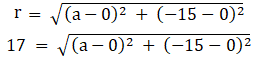172 =a2 + (-15)2

289 = a2 +225

a2 =64

|a| = √64

|a| = 8

a = ±8 …. (1)

We have got the centreat (±8, – 15) and having radius 17 units.

We know that theequation of the circle with centre (p, q) and having radius ‘r’ is given by: (x– p)2 + (y – q)2 = r2

Now by substitutingthe values in the equation, we get

(x ± 8)2 +(y – 15)2 = 172

x±16x + 64 + y2 – 30y + 225 = 289

x2 + y±16x – 30y = 0.

The equation of thecircle is x2 + y± 16x – 30y = 0.

Question 8 : Find the equation of the circle which has its centre at the point (3, 4) and touches the straight line 5x + 12y – 1 = 0.

It is given that weneed to find the equation of the circle with centre (3, 4) and touches thestraight line 5x + 12y – 1 = 0.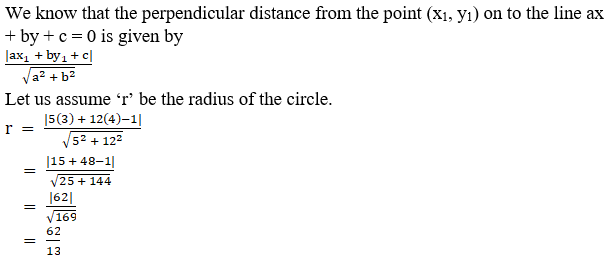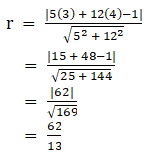We have a circle withcentre (3, 4) and having a radius 62/13.

We know that theequation of the circle with centre (p, q) and having radius ‘r’ is given by: (x– p)2 + (y – q)2 = r2

Now by substitutingthe values in the equation, we get

(x – 3)2 +(y – 4)2 = (62/13)2

x2 –6x + 9 + y2 – 8y + 16 = 3844/169

169x2 +169y2 – 1014x – 1352y + 4225 = 3844

169x2 +169y2 – 1014x – 1352y + 381 = 0

The equation of thecircle is 169x2 + 169y2 – 1014x – 1352y + 381 =0.

Question 9 : Find the equation of the circle which touches the axes and whose centre lies on x – 2y = 3.

Let us assume thecircle touches the axes at (a, 0) and (0, a) and we get the radius to be |a|.

We get the centre ofthe circle as (a, a). This point lies on the line x – 2y = 3

a – 2(a) = 3

-a = 3

a = – 3

Centre = (a, a) = (-3,-3) and radius of the circle(r) = |-3| = 3

We have circle withcentre (-3, -3) and having radius 3.

We know that theequation of the circle with centre (p, q) and having radius ‘r’ is given by: (x– p)2 + (y – q)2 = r2

Now by substitutingthe values in the equation, we get

(x – (-3))2 +(y – (-3))2 = 32

(x + 3)2 +(y + 3)2 = 9

x2 +6x + 9 + y2 + 6y + 9 = 9

x2 + y2 +6x + 6y + 9 = 0

The equation of thecircle is x2 + y2 + 6x + 6y + 9 = 0.

Question 10 : A circle whose centre is the point of intersection of the lines 2x – 3y + 4 = 0 and 3x + 4y – 5 = 0 passes through the origin. Find its equation.

Answer 10 : It is given that the circle has the centre at the intersection point ofthe lines 2x – 3y + 4 = 0 and 3x + 4y – 5 = 0 and passes through the origin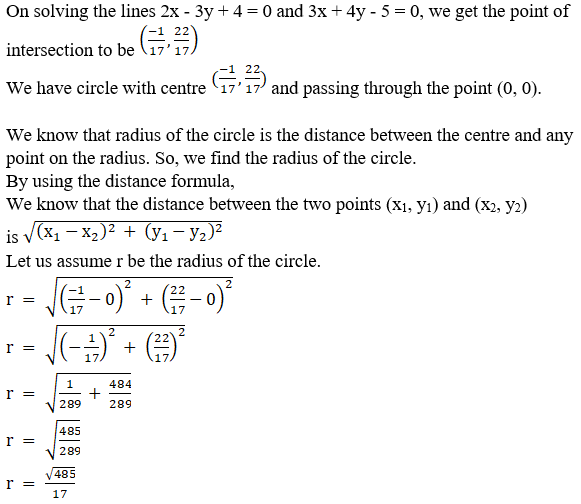We know that theequation of the circle with centre (p, q) and having radius ‘r’ is given by: (x– p)2 + (y – q)2 = r2

Now by substitutingthe values in the equation, we get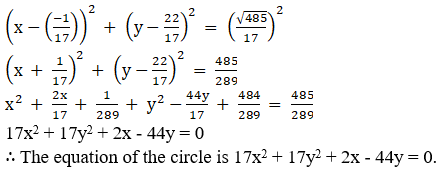Todays Deals### RD Chapter 24- The Circle Ex-24.1 Contributorskrishan

Name:
Email:

# Latest News# 9000 interview questions in different categories